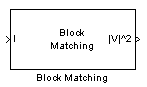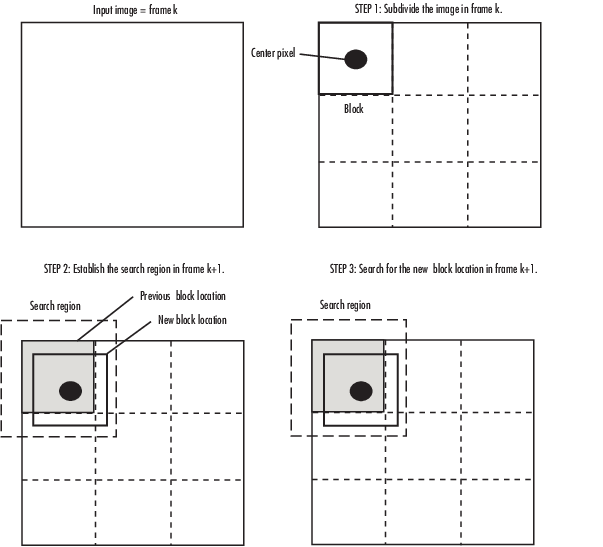# Block Matching

Estimate motion between images or video frames

• Library:
• Computer Vision Toolbox / Analysis & Enhancement

•## Description

The Block Matching block estimates motion between two images or two video frames by comparing blocks of pixels. The block matches the block of pixels between frames by moving the block of pixels over a search region.

## Ports

### Input

expand all

Image or video frame, specified as a scalar, vector, or matrix. If the Estimate motion between parameter is set to Two images, the name of this port changes to I1.

Data Types: `single` | `double` | `int8` | `int16` | `int32` | `int64` | `uint8` | `uint16` | `uint32` | `uint64` | `fixed point`

Image or video frame, specified as a scalar, vector, or matrix.

#### Dependencies

To enable this port, set the Estimate motion between parameter to `Two images`.

Data Types: `single` | `double` | `int8` | `int16` | `int32` | `int64` | `uint8` | `uint16` | `uint32` | `uint64` | `fixed point`

### Output

expand all

Velocity magnitudes, returned as a scalar, vector, or matrix.

Data Types: `single` | `double` | `int8` | `int16` | `int32` | `int64` | `uint8` | `uint16` | `uint32` | `uint64` | `fixed point`

Velocity components in complex form, returned as a scalar, vector, or matrix.

#### Dependencies

To expose this port, set the Velocity output parameter to ```Horizontal and vertical components in complex form```.

Data Types: `single` | `double` | `int8` | `int16` | `int32` | `int64` | `uint8` | `uint16` | `uint32` | `uint64` | `fixed point`

## Parameters

expand all

Main Tab

Specify whether to estimate the motion between two images or two video frames. Select `Two images` to estimate the motion between two images. Select `Current frame and N-th frame back` to estimate the motion between two video frames that are N frames apart.

#### Dependencies

Set this parameter to `Current frame and N-th frame back` to expose the N parameter.

Specify a scalar value that represents the number of frames between the reference frame and the current frame.

#### Dependencies

To enable this parameter, set the Estimate motion between parameter to `Current frame and N-th frame back`.

Specify how the block locates the block of pixels in the current frame and the next frame that best matches the block of pixels.

• `Exhaustive` — The block selects the location of the block of pixels in the next frame by moving the block over the search region one pixel at a time. This process is computationally expensive.

• `Three-step` — The block searches for the block of pixels in the next frame that best matches the block of pixels in the current frame by using a steadily decreasing step size. The block begins with a step size approximately equal to half the maximum search range. In each step, the block compares the central point of the search region to eight search points located on the boundaries of the region and moves the central point to the search point whose values is the closest to that of the central point. The block then reduces the step size by half, and begins the process again. This option is less computationally expensive, though it might not find the optimal solution.

Specify how the block measures the similarity of the block of pixels in the current frame to the block of pixels in the next frame.

If you select `Mean square error (MSE)`, the block estimates the displacement of the center pixel of the block as the $\left({d}_{1},{d}_{2}\right)$ values that minimize this MSE equation:

`$MSE\left({d}_{1},{d}_{2}\right)=\frac{1}{{N}_{1}×{N}_{2}}\sum _{\left({n}_{1},{n}_{2}\right),}\sum _{\in B}{\left[s\left({n}_{1},{n}_{2},k\right)-s\left({n}_{1}+{d}_{1},{n}_{2}+{d}_{2},k+1\right)\right]}^{2}$`

In the previous equation, B is an ${N}_{1}×{N}_{2}$ block of pixels, and s(x,y,k) denotes a pixel location at (x,y) in frame k.

If you select `Mean absolute difference (MAD)`, the Block Matching block estimates the displacement of the center pixel of the block as the $\left({d}_{1},{d}_{2}\right)$ values that minimize this MAD equation:

`$MAD\left({d}_{1},{d}_{2}\right)=\frac{1}{{N}_{1}×{N}_{2}}\sum _{\left({n}_{1},{n}_{2}\right),}\sum _{\in B}|s\left({n}_{1},{n}_{2},k\right)-s\left({n}_{1}+{d}_{1},{n}_{2}+{d}_{2},k+1\right)|$`

Specify the size of the block of pixels.

Specify the overlap (in pixels) of two subdivisions of the input image.Specify the maximum number of pixels any center pixel in a block of pixels might move from image to image or frame to frame. The block uses this value to determine the size of the search region.

Specify the output. If you select `Magnitude-squared`, the block outputs an optical flow matrix where each element is in the form u2+v2. If you select ```Horizontal and vertical components in complex form```, the block outputs an optical flow matrix where each element is in the form $u+jv$. The real part of each value is the horizontal velocity component and the imaginary part of each value is the vertical velocity component.

Data Types Tab

For details on the fixed-point block parameters, see Specify Fixed-Point Attributes for Blocks.

## Block Characteristics

 Data Types `double` | `fixed point` | `integer` | `single` Multidimensional Signals `no` Variable-Size Signals `no`

expand all